# How to filter out noise in your DC/DC design

After establishing a baseline on power-supply noise, let’s focus on post-filtering strategies of DC/DC converters while driving an analog-to-digital converter (ADC). For continuity with previous posts, I will again use the ADC3444, a quad, 14-bit, 125MSPS pipeline ADC with low-voltage differential signaling (LVDS) output.

In my “Using a DC/DC converter to power an ADC” post, I established a baseline using a battery for power followed by two single-channel low-dropout  voltage regulators (LDOs) using TI’s TPS7A47.The idea here is to isolate the digital VDD (DVDD) supply of the ADC3444 from the analog VDD (AVDD) supply. But it’s also possible to achieve the same level of performance of the TPS7A47 reference while using smaller-size, higher-efficiency solutions.

The ADC3444 is powered by a TPS54120 DC/DC converter and generates an undesired signal on both the AVDD or DVDD pins that need to be rejected. Figures 1 and 2 illustrate the undesired signals coming from the power supplies. These signals (Figure 1) are originating as the switching frequency of the DC/DC and are modulated by the ADC clock-phase noise.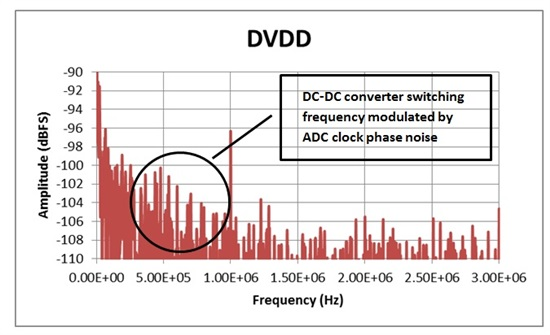Figure 1: Undesired DVDD signal generated in the power supply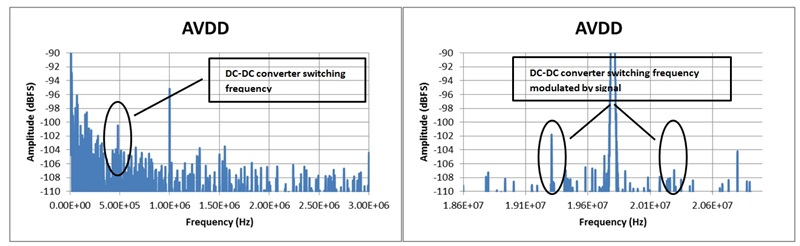Figure 2: Undesired AVDD signal generated in the power supply (a) at DC and (b) around a single tone

The first approach is to have the signal filtered in the digital domain. To implement this approach successfully, undesirable signals must be deterministic. For DVDD, the undesirable signal is a modulation of the phase noise with the switching frequency; thus a wide frequency band needs to be eliminated. For AVDD, the undesirable signal around the single tone, see Figure 2, is a lot harder to remove. Thus, digitally filtering this undesirable signal does not seem the best approach. It is therefore critical to remove undesirable signals at their source.

The two options to reduce noise originating in DC/DC converters are π-filters and active devices such as LDOs or power filters.

The π-filter is composed of two capacitors separated by an inductor or ferrite bead. Here, a Murata ferrite bead is selected. The BLM41PG102 has 1kΩ impedance at 100MHz. Figure 3 shows the impedance characteristic.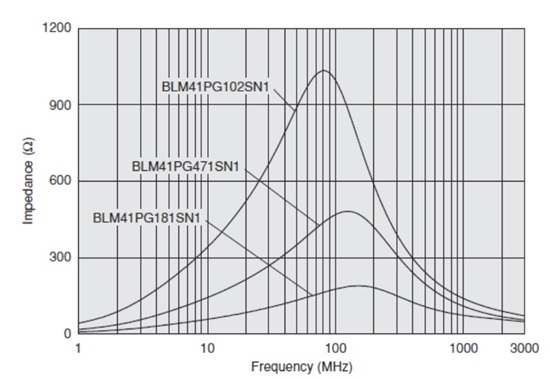Figure 3: Murata BLM41PG ferrite-bead impedance versus frequency plot

Looking at the AVDD supply, the ADC3444 has 13 AVDD power pins, each bypassed by a 0.1µF capacitor. Importing the 0.1µF model from the Kemet library and plotting the π-filter frequency response, you have the following curve, see Figure 4.Figure 4: Filter-frequency response for various capacitor temperatures

This filter is essentially behaving as a second-order response with a -3dB point at . Integrating the area below the ideal capacitor filter and dividing it by f-3dB gives you the relationship between the -3dB bandwidth and the equivalent brick-wall filter bandwidth used for noise-power calculations (Figure 5).

This plot only provides variations of the filter noise-power bandwidth (NPBW) and indicates that most of the NPBW is contributed below 1MHz.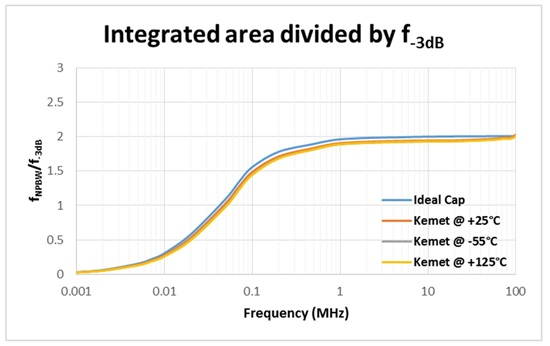Figure 5: Noise-power bandwidth divided by -3dB bandwidth versus frequency

Now that I’ve established both the attenuation that the undesirable signals will see prior to reaching the ADC and the effective NPBW, to help limit the thermal noise of the power supply, let’s go back to the ADC3444 power-supply rejection ratio (PSRR) on AVDD. The π-filter, made of the BLM41PG102 and thirteen 0.1µF capacitors, improves the PSRR of the ADC by 36dB at 500kHz and will attenuate the thermal noise by an optimistic . (The  ratio is optimistic because the largest part of the thermal noise is due to  noise.) Root mean square (RMS) thermal noise is typically measured over the 10Hz-to-100kHz bandwidth, and the NPBW of the π-filter is 64kHz.

The ferrite bead should be sufficient to eliminate the undesired signals, but it is rare to use a 3A DC/DC converter or even a 1.2A DC/DC converter solely to operate a 100mA load. If the DC/DC converter was shared by multiple loads, crosstalk between loads would then become the main problem to resolve.

Let’s now turn our attention to active devices.

LDOs regulate the output voltage to a given accuracy, typically 1 to 3 percent. They will have a high PSRR at DC and decrease as frequency increases. Figure 6 shows the TPS7A81 1A low-noise LDO implemented in an adjustable configuration with the output voltage set by R1 and R2. A feed-forward capacitor (CBYPASS) is implemented to limit the noise and also improve the output voltage transient. The noise reduction capacitor (CNR) reduces the noise originating in the on-chip voltage reference. Input and output capacitors are present.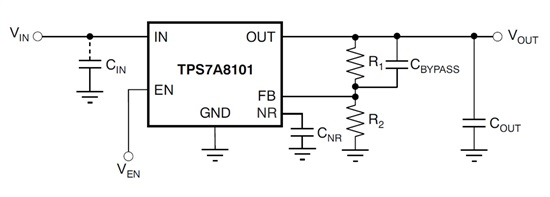Figure 6: LDO architecture

In contrast, a power filter regulates the voltage differential between the input and the output. This architecture has no PSRR at DC, and the PSRR increases with frequency. Figure 7 shows the TPS7A35 1A low-noise power filter. Since the voltage drop between input and output is regulated, the voltage drop is set with a single resistor (RNR). Noise reduction (CNR) and the input and output capacitor are present as well. This solution can be attractive for its small PCB footprint.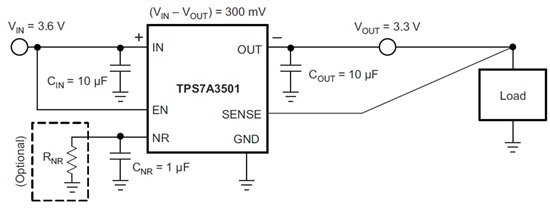Figure 7: Power filter architecture

Table 1 lists the pros and cons of each solution.

Table 1: Post-filtering implementation comparison

The attenuation provided to the DC/DC ripple by the PSRR for any solution above will behave as Equation 1: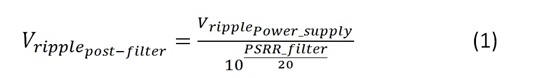And the thermal noise as Equation 2: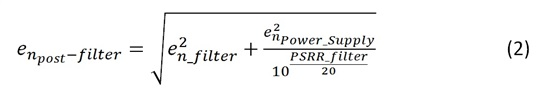In this post, I’ve looked at various post-filtering strategies for the DC/DC converter and developed an analytical approach to compare one solution versus another. In the final installment of this series, I will put it all together and implement a low-noise, high-PSRR power supply and evaluate ADC performance.

In case you missed any other posts in this series, read my other blogs on creating a power supply for ADCs.